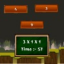Hlavní navigace

#iDigitalMaths 1.1

This free iDigitalMaths helps player to develop mathematical, tactile and fine motor skills while playing different levels. Player can solve mathematical equations of the three numbers in this app. Player have functionality to choose number digits in this app.

How to play?
1. Addition:- Player have one three number addition equation and the player can solve that equation and then choose right answer form the given options. Player have right and wrong answer count into the screen. it also display right answer suggestion when choose wrong answer.
2. Subtraction:- Player have one three number subtraction equation and the player can solve that equation and then choose right answer form the given options. Player have right and wrong answer count into the screen. it also display right answer suggestion when choose wrong answer.
3. Multiplication:- Player have one three number multiplication equation and the player can solve that equation and then choose right answer form the given options. Player have right and wrong answer count into the screen. it also display right answer suggestion when choose wrong answer.
4. Division:- Player have one three number division equation and the player can solve that equation and then choose right answer form the given options. Player have right and wrong answer count into the screen. it also display right answer suggestion when choose wrong answer.

## Celkové hodnocení

Průměr hodnocení
3

Pro hodnocení programu se prosím nejprve

## Souhrnné informace o iDigitalMaths

1.1

ne

24,02 MB

• Angličtina
• #### Staženo

0× celkem
0× tento měsíc

15. 5. 2020

## Něco jsme propásli?

Dejte nám vědět. Upozornit redakci Stahuj
Velice děkujeme za Vaše podněty